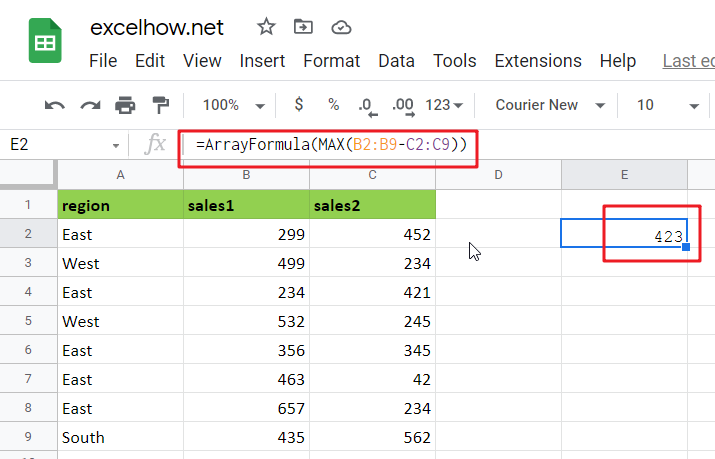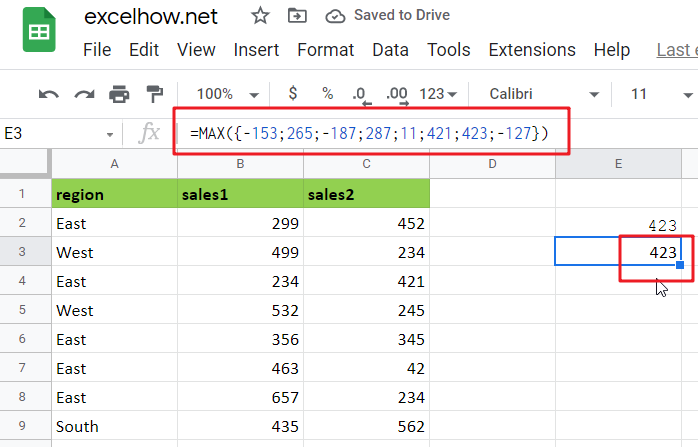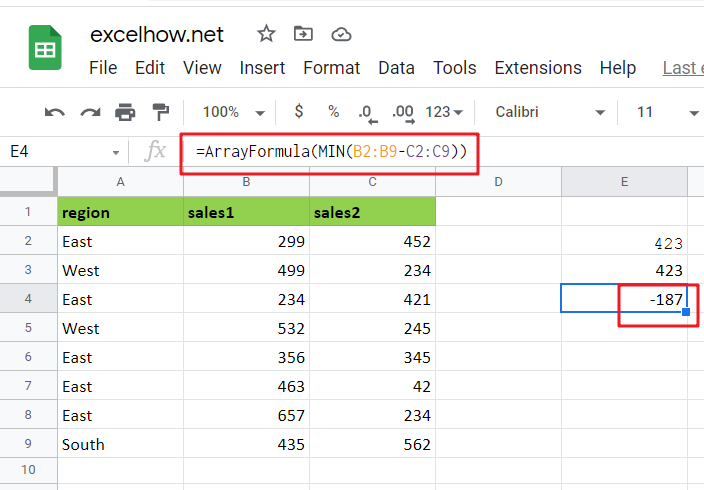# Basic Array Formula With Examples in Google Sheets

Are you searching for an article for getting the basic array formula with different examples for better understanding? Then congratulations because you have just landed on the right article. In this article, you would get to know the basic array formula in google sheets and the use of this formula in different examples with a brief explanation.

### General Formula in Google Sheets

The basic array formula is given as follows:

{=MAX(range1-range2)}

### Syntax Explanation

• Minus Operator (-): This symbol is used to subtract any two values.
• Parenthesis (): The main function of this symbol is to group the elements.
• range1: This is the first input range in the Google sheet.
• Range2: This refers to the second input range.
• MAX: The MAX Function returns the largest numeric value from the range of input values.
• MIN: The MIN Function returns the smallest numeric value from a range of input values.

## Explanation

In Google Sheets, an Array Formula allows you to perform complex calculations on one or more value sets. The result could be a single cell or an array. An array is simply a list or range of values, but an Array Formula is a particular type of formula that must be entered by pressing Ctrl+Shift+Enter after that, the formula would be displayed in the formula bar surrounded by curly brackets {=…}

Array formulas are commonly used for data analysis, conditional sums and lookups, linear algebra, matrix math and manipulation, and many other tasks. A new Google Sheets user may come across array formulas in other people’s spreadsheets, but creating array formulas is typically an intermediate-to-advanced topic.

An array formula is commonly used to calculate the maximum or minimum change in a data set without using a helper column. In the example, the formula in E2 is mentioned as follows:

`=MAX(B2:B9-C2:C9)`

#This array formula must be entered with control + shift + enter.

This page’s example shows a simple array formula. Working from the inside out, the expression:

=B2:B9-C2:C9

As a result, an array of seven values is returned:

`{-153;265;-187;287;11;421;423;-127}`

#Each number in the array results from subtracting the “low” from the “high” in each of the seven rows of data. This array is returned to the MAX function:

=MAX({-153;265;-187;287;11;421;423;-127})

#And MAX returns the maximum value in the array, which is 423.

To return the smallest change in the data, substitute the MIN function for the MAX function:

`=MIN(B2:B9-C2:C9)`

#As before, this is an array formula that must be entered with control + shift + enter.

## More Examples For Better Understanding in Google Sheets

For basic array formulas in Google Sheets, use the steps below.

In the following example, you must create a sample data set in Google Sheets.

Then, in the formula bar, use the following formula to count the maximum change in a data set.

=MAX(B2:B9-C2:C9)

After pressing Ctrl+Shift+Enter, you will see the MAX value in cell E2.

Then, in the formula bar, use the following formula to count the minimum change in a data set.

=MIN(B2:B9-C2:C9)

Finally, after pressing Ctrl+Shift+Enter, you will see the result for the MIN in cell F2.

## More On Array Formulas

To understand array formulas, you must first learn to investigate the results of various operations within a formula as Google Sheets evaluates it. You must understand how to use the F9 key to debug a formula and how to use Google Sheets’s Evaluate Feature.